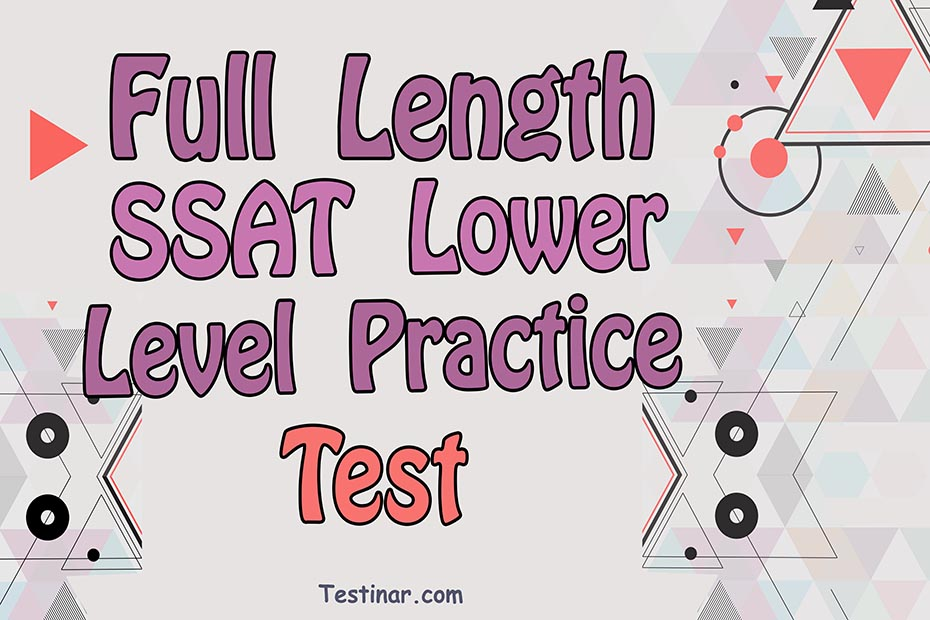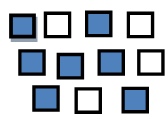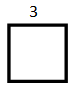## Full Length SSAT Lower Level Practice Test

If you want to prepare for the SSAT Lower Level Practice Test? It’s time to taking a Full-length SSAT Lower Level Practice Test. It is the best way to simulate test day. To challenge your skills and simulate the full length SSAT Lower Level Practice Test day experience, score your tests using the answer keys.

### 5 SSAT Lower Level Math Practice Tests

$21.99$16.99
23% Off*

Extra Practice to Help Achieve an Excellent Score

SSAT Lower LevelQuantitative Practice Test 3

30 questions
Total time for this test: 30 Minutes
You may NOT use a calculator for this test.

1- If $$\frac{1}{5}$$ of a number is greater than $$6$$, the number must be …..

(A) $$24$$
(B) $$12$$
(C) $$30$$
(D) $$20$$
(E) $$18$$
2- In the following figure, the shaded squares are what fractional part of the whole set of squares?(A) $$\frac{7}{11}$$
(B) $$\frac{2}{11}$$
(C) $$\frac{2}{7}$$
(D) $$\frac{5}{11}$$
(E) $$\frac{4}{5}$$
3- If $$6 \ × \ (M \ + \ N)=24$$ and $$M$$ is greater than $$0$$, then $$N$$ could Not be …..
(A) $$6$$
(B) $$4$$
(C) $$10$$
(D) $$7$$
(E) $$5$$
4- Which of the following is greater than $$\frac{6}{4}$$?
(A) $$\frac{1}{2}$$
(B) $$\frac{4}{5}$$
(C) $$\frac{5}{3}$$
(D) $$\frac{7}{3}$$
(E) $$\frac{2}{5}$$
5- Which of the following is closest to $$7.06$$?
(A) $$5$$
(B) $$4.5$$
(C) $$7.5$$
(D) $$6$$
(E) $$7$$
6- In the multiplication bellow, $$A$$ represents which digit?
$$12 \ × \ 6 \ A \ 2=7,344$$
(A) $$1$$
(B) $$5$$
(C) $$8$$
(D) $$4$$
(E) $$3$$
7- $$9.8 \ - \ 5.02$$ is closest to which of the following.
(A) $$11$$
(B) $$15$$
(C) $$4.05$$
(D) $$7.32$$
(E) $$4.78$$
8- If $$N$$ is an even number, which of the following is always an odd number?
(A) $$\frac{N}{2}$$
(B) $$N \ + \ 4$$
(C) $$2 \ N$$
(D) $$2 \times N \ + 2$$
(E) $$N \ + 1$$
9- Numbers x and y are shown above. How many times larger is the value of digit $$6$$ in the number $$x$$, than the value of digit $$6$$ in the number $$y$$?
$$x=3,267 \ \ y=465$$

(A) $$1$$
(B) $$10$$
(C) $$100$$
(D) $$1000$$
(E) $$10,000$$
10- At a Zoo, the ratio of lions to tigers is $$9$$ to $$6$$. Which of the following could NOT be the total number of lions and tigers in the zoo?
(A) $$35$$
(B) $$78$$
(C) $$55$$
(D) $$65$$
(E) $$70$$
11- If $$24$$ can be divided by both $$6$$ and $$x$$ without leaving a remainder, then $$24$$ can also be divided by which of the following?
(A) $$x \ +\ 6$$
(B) $$2 \ x \ - \ 4$$
(C) $$x \ +\ 1$$
(D) $$2 \ x \ × \ 2 \ - \ 5$$
(E) $$4 \ x \ - \ 7$$
12- If $$4$$ added to a number, the sum is $$15$$. If the same number added to $$10$$, the answer is:
(A) $$35$$
(B) $$22$$
(C) $$14$$
(D) $$40$$
(E) $$21$$
13- What is the Area of the square shown in the following square?(A) $$4$$
(B) $$7$$
(C) $$4$$
(D) $$9$$
(E) $$10$$
14- Use the equations below to answer the question:
$$x \ - \ 10=12$$
$$12 \ + \ y=27$$
What is the value of $$x \ + \ y$$ ?
(A) $$12$$
(B) $$17$$
(C) $$8$$
(D) $$18$$
(E) $$11$$
15- $$\frac{3 \ + \ 3 \ + \ 5 \ × \ 2 \ + \ 2}{4 \ + \ 2}=$$?
(A) $$4$$
(B) $$8$$
(C) $$\frac{4}{5}$$
(D) $$\frac{2}{7}$$
(E) $$5$$
16- Which of the following expressions has the same value as $$\frac{2}{3} \ × \ \frac{4}{2}$$?
(A) $$\frac{3 \times \ 2}{5}$$
(B) $$\frac{4 \times \ 2}{5}$$
(C) $$\frac{2 \times \ 2}{5}$$
(D) $$\frac{3 \times \ 6}{5}$$
(E) $$\frac{2 \times \ 5}{5}$$
17- If a triangle has a base of $$4$$ cm and a height of $$7$$ cm, what is the area of the triangle?
(A) $$12$$ cm$$^2$$
(B) $$14$$ cm$$^2$$
(C) $$8$$ cm$$^2$$
(D) $$15$$ cm$$^2$$
(E) $$10$$ cm$$^2$$
18- When $$4$$ is added to three times number $$N$$, the result is $$25$$. Then $$N$$ is ….
(A) $$3$$
(B) $$5$$
(C) $$7$$
(D) $$4$$
(E) $$15$$

### 5 Full Length SSAT Lower Level Math Practice Tests

$16.99$11.99
29% Off*

The Practice You Need to Ace the SSAT Lower Level Math Test

19- What is the next number in this sequence?  $$3, \ 6, \ 10, \ 15, \ 21,$$ …
(A) $$28$$
(B) $$26$$
(C) $$30$$
(D) $$29$$
(E) $$35$$
20- At noon, the temperature was $$12$$ degrees. By midnight, it had dropped another $$22$$ degrees. What was the temperature at midnight?
(A) $$8$$ degrees above zero
(B) $$8$$ degrees below zero
(C) $$10$$ degrees below zero
(D) $$7$$ degrees above zero
(E) $$5$$ degrees above zero
21- Which formula would you use to find the area of a square?
(A) length $$×$$ width $$×$$ height
(B) $$\frac{1}{2}$$ base $$\times$$ heigth
(C) length $$\times$$ width
(D) $$\frac{1}{2}$$ (length $$\times$$ width $$\times$$ heigth)
(E) side $$\times$$ side
22- If there are $$6$$ red balls and $$10$$ blue balls in a basket, what is the probability that Lucy will pick out a red ball from the basket?
(A) $$\frac{3}{8}$$
(B) $$\frac{3}{4}$$
(C) $$\frac{4}{5}$$
(D) $$\frac{2}{3}$$
(E) $$\frac{6}{10}$$
23- What is $$20\%$$ of $$500$$?
(A) $$60$$
(B) $$25$$
(C) $$300$$
(D) $$25$$
(E) $$50$$
24- What is the average of the following numbers?  $$3, \ 6, \ 17, \ 28, \ 45$$
(A) $$19$$
(B) $$22$$
(C) $$19.8$$
(D) $$19.5$$
(E) $$24$$
25- How many lines of symmetry does an equilateral triangle have?
(A) $$4$$
(B) $$1$$
(C) $$5$$
(D) $$3$$
(E) $$2$$
26- If all the sides in the following figure are of equal length and length of one side is $$5$$, what is the perimeter of the figure?(A) $$25$$
(B) $$30$$
(C) $$18$$
(D) $$40$$
(E) $$28$$
27- If $$N=4$$ and $$\frac{48}{N} \ + \ 5= \Box$$, then $$\Box=$$ …..
(A) $$24$$
(B) $$8$$
(C) $$17$$
(D) $$10$$
(E) $$22$$
28- Which of the following statement is False?
(A) $$3 \ × \ 2=10$$
(B) $$(5 \ + \ 2) \ × \ 2=14$$
(C) $$9 \ ÷ \ (6 \ - \ 3)=3$$
(D) $$4\ × \ (5 \ - \ 2)=12$$
(E) $$(8 \ + \ 4) \ × \ 10=120$$
29- Four people can paint $$4$$ houses in $$10$$ days. How many people are needed to paint $$8$$ houses in $$5$$ days?
(A) $$8$$
(B) $$14$$
(C) $$17$$
(D) $$16$$
(E) $$13$$
30- $$\frac{6}{2} \ - \ \frac{5}{2}=$$ ?
(A) $$0.35$$
(B) $$0.25$$
(C) $$0.5$$
(D) $$0.2$$
(E) $$0.75$$
 1- Choice C is correct The correct answer is Greater than $$30$$If $$\frac{1}{5}$$ of a number is greater than $$6$$, the number must be greater than $$30$$. $$\frac{1}{5} \ x \ > \ 6→$$ multiply both sides of the inequality by $$6$$,then: $$x \ > \ 30$$ 2- Choice A is correct The correct answer is $$\frac{7}{11}$$There are $$11$$ squares and $$7$$ of them are shaded.Therefore, $$7$$ out of $$11$$ or $$\frac{7}{11}$$ are shaded. 3- Choice B is correct The correct answer is $$4$$$$6 \ × \ (M \ + \ N)=24$$, then $$M \ + \ N=4. \ M \ > \ 0→N$$ could not be $$4$$. 4- Choice D is correct The correct answer is $$\frac{7}{3}$$$$\frac{6}{4}=1.5$$, the only option that is greater than $$1.5$$ is $$\frac{7}{3}$$.$$\frac{5}{2}=2.33 ,2.33 \ > \ 1.5$$ 5- Choice E is correct The correct answer is $$7$$The closest to $$7.06$$ is $$7$$ in the options provided. 6- Choice A is correct The correct answer is $$1$$$$A$$ represents digit $$1$$ in the multiplication. $$12 \ × \ 612=7,344$$ 7- Choice E is correct The correct answer is $$4.78$$$$8.9 \ - \ 4.08=4.78$$, which is closest to $$4.78$$ 8- Choice E is correct The correct answer is $$N \ + \ 1$$$$N$$ is even. Let’s choose $$2$$ and $$4$$ for $$N$$.Now, let’s review the options provided. A. $$\frac{N}{2}=\frac{2}{2}=1, \ \frac{N}{2}=\frac{4}{2}=2$$,                                                      One result is odd and the other one is even.B. $$N \ + \ 4=2 \ + \ 4=6, \ 4 \ + \ 4=8$$                                        Both results are even.C. $$2 \ N=2 \ × \ 2=4, \ 4 \ × \ 2=8$$                                              Both results are even.D. $$(2 \ × \ N) \ + \ 2=(2 \ × \ 2) \ + \ 2=6, \ (4 \ × \ 2) \ + \ 2=10$$ Both results are even.E. $$N \ + \ 1=2 \ + \ 1=3, \ 4 \ + \ 1=5$$                                        Both results are odd. 9- Choice A is correct The correct answer is $$1$$The value of digit $$6$$ in both numbers $$x$$ and $$y$$ are in the tens place.Therefore, they have the same value. 10- Choice B is correct The correct answer is $$78$$The ratio of lions to tigers is $$9$$ to $$6$$ or $$3$$ to $$2$$ at the zoo.Therefore, total number of lions and tigers must be divisible by $$5$$. $$3 \ + \ 2=5$$From the numbers provided, only $$78$$ is not divisible by $$5$$. 11- Choice B is correct The correct answer is $$2 \ x \ - \ 4$$$$24=x \ × \ 6→x=24 \ ÷ \ 6=4$$$$x$$ equals to $$4$$.Let’s review the options provided: A. $$x \ +\ 6→4 \ + \ 4=10$$                          $$24$$ is not divisible by $$10$$.B. $$2 \ x \ - \ 4→2 \ × \ 4 \ - \ 4=4$$                 $$24$$ is  divisible by $$4$$.C. $$x \ +\ 1→4 \ + \ 1=5$$                            $$24$$ is not divisible by $$5$$.D. $$2 \ x \ × \ 2 \ - \ 5→16 \ - \ 5=11$$            $$24$$ is not divisible by $$11$$.E. $$4 \ x \ - \ 7=16 \ - \ 7=9$$                         $$24$$ is not divisible by $$9$$. 12- Choice E is correct The correct answer is $$21$$$$4 \ + \ x=15→$$$$x=11→$$$$11 \ + \ 10=21$$ 13- Choice D is correct The correct answer is $$9$$Area of a square $$=$$ (one side) $$×$$ (one side)$$3 \ × \ 3=9$$ 14- Choice B is correct The correct answer is $$17$$$$x \ - \ 10=12→x=2$$$$12 \ + \ y=27→y=15$$$$x \ + \ y=2 \ + \ 15=17$$ 15- Choice A is correct The correct answer is $$4$$$$\frac{3 \ + \ 3 \ + \ 5 \ × \ 2 \ + \ 2}{4 \ + \ 2}=\frac{24}{6}=4$$ 16- Choice C is correct The correct answer is $$\frac{2 \ × \ 2}{5}$$$$\frac{2}{3} \ × \ \frac{4}{2}=\frac{8}{10}=\frac{4}{5}$$ $$\frac{2 \ × \ 2}{5}=\frac{4}{5}$$ 17- Choice B is correct The correct answer is $$14$$ cm$$^2$$Area of a triangle $$= \frac{1}{2} \ ×$$ (base) $$×$$ (height) $$= \frac{1}{2} \ × \ 4 \ × \ 7=14$$ cm$$^2$$ 18- Choice C is correct The correct answer is $$7$$$$4 \ + \ 3 \ N=25→$$$$3 \ N=25 \ - \ 4=21→$$$$N=7$$ 19- Choice A is correct The correct answer is $$28$$$$3 \ + \ 3=6→$$$$6 \ +\ 4=10→$$$$10 \ + \ 5=15→$$$$15 \ + \ 6=21→$$$$21 \ + \ 7=28$$ 20- Choice C is correct The correct answer is $$10$$ degrees below zero$$12 \ -\ 22= \ - \ 10$$The temperature at midnight was $$10$$ degrees below zero. 21- Choice E is correct The correct answer is side $$×$$ side area of a square $$=$$ side $$×$$ side 22- Choice A is correct The correct answer is $$\frac{3}{8}$$There are $$6$$ red ball and $$16$$ are total number of balls.Therefore, probability that Lucy will pick out a red ball from the basket is $$6$$ out of $$16$$ or $$\frac{6}{6 \ + \ 10}=\frac{6}{16}=\frac{3}{8}$$. 23- Choice E is correct The correct answer is $$50$$$$20$$ percent of $$500 = 20\%$$ of $$500 = \frac{10}{100} \ × \ 500=50$$ 24- Choice C is correct The correct answer is $$19.8$$average $$=\frac{sum \ of \ all \ numbers}{number \ of \ numbers}=\frac{3 \ + \ 6 \ + \ 17 \ + \ 28 \ + \ 45}{5}=19.8$$ 25- Choice D is correct The correct answer is $$3$$An equilateral triangle has $$3$$ lines of symmetry. 26- Choice B is correct The correct answer is $$30$$The shape has $$6$$ equal sides.And is side is $$5$$.Then, the perimeter of the shape is:$$5 \ × \ 6=30$$ 27- Choice C is correct The correct answer is $$17$$$$N=4$$, then:$$\frac{48}{4} \ + \ 5=12 \ + \ 5=17$$ 28- Choice A is correct The correct answer is $$3 \ × \ 2=10$$Let’s review the options provided: A. $$3 \ × \ 2=10$$                                                     This is NOT true!B. $$(5 \ + \ 2) \ × \ 2=14$$                                          This is true!C. $$9 \ ÷ \ (6 \ - \ 3)=3→9 \ ÷\ 3=3$$                   This is true!D. $$4\ × \ (5 \ - \ 2)=12→4 \ × \ 3=12$$               This is true!E. $$(8 \ + \ 4) \ × \ 10=120→12 \ ×\ 10=120$$      This is true! 29- Choice D is correct The correct answer is $$16$$Four people can paint $$4$$ houses in $$10$$ days.It means that for painting $$8$$ houses in $$10$$ days we need $$8$$ people.To paint $$8$$ houses in $$5$$ days, $$16$$ people are needed. 30- Choice C is correct The correct answer is $$0.5$$$$\frac{6}{2} \ - \ \frac{5}{2}=\frac{1}{2}=0.5$$

### 5 SSAT Lower Level Math Practice Tests

$21.99$16.99
23% Off*

Extra Practice to Help Achieve an Excellent Score

### Practice Test 1

Simulate test day with an official practice test. Then, score your test. The answers come with explanations so you can learn from your mistakes.

### Practice Test 2

Simulate test day with an official practice test. Then, score your test. The answers come with explanations so you can learn from your mistakes.

### CLEP College Mathematics Study Guide

$20.99$15.99

### TABE 11 & 12 Math in 10 Days

$24.99$13.99

### SHSAT Mathematics Formulas

$6.99$6.99

### CLEP College Algebra Exercise Book 2020-2021

$23.99$18.99